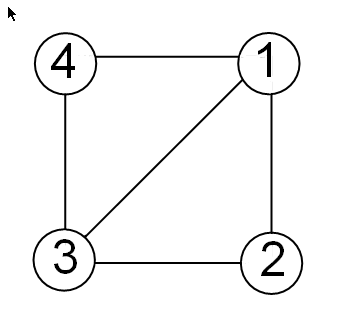Documentation

# unmesh

Convert edge matrix to coordinate and Laplacian matrices

## Syntax

```[L,XY] = unmesh(E) ```

## Description

`[L,XY] = unmesh(E)` returns the Laplacian matrix `L` and mesh vertex coordinate matrix `XY` for the `M`-by-4 edge matrix `E`. Each row of the edge matrix must contain the coordinates ```[x1 y1 x2 y2]``` of the edge endpoints.

## Input Arguments

 `E` M-by-4 edge matrix `E`.

## Output Arguments

 `L` Laplacian matrix representation of the graph. `XY` Mesh vertex coordinate matrix.

## Examples

Take a simple example of a square with vertices at (1,1), (1,–1),(–1,–1), and (–1,1), where the connections between vertices are the four perpendicular edges of the square plus one diagonal connection between (–1, –1) and (1,1).The edge matrix `E` for this graph is:

```E=[1 1 1 -1; % edge from 1 to 2 1 -1 -1 -1; % edge from 2 to 3 -1 -1 -1 1; % edge from 3 to 4 -1 -1 1 1; % edge from 3 to 1 -1 1 1 1] % edge from 4 to 1```
Use `unmesh` to create the output matrices,
```[A,XY]=unmesh(E); 4 vertices: 4/4```
The Laplacian matrix is defined as

`unmesh` returns the Laplacian matrix `L` in sparse notation.

```L L = (1,1) 3 (2,1) -1 (3,1) -1 (4,1) -1 (1,2) -1 (2,2) 2 (4,2) -1 (1,3) -1 (3,3) 2 (4,3) -1 (1,4) -1 (2,4) -1 (3,4) -1```
To see `L` in regular matrix notation, use the `full` command.
```full(L) ans = 3 -1 -1 -1 -1 2 0 -1 -1 0 2 -1 -1 -1 -1 3 ```
The mesh coordinate matrix `XY` returns the coordinates of the corners of the square.
```XY XY = -1 -1 -1 1 1 -1 1 1```